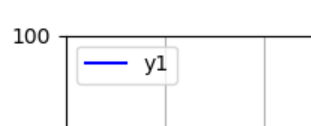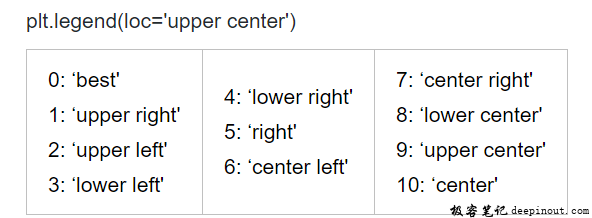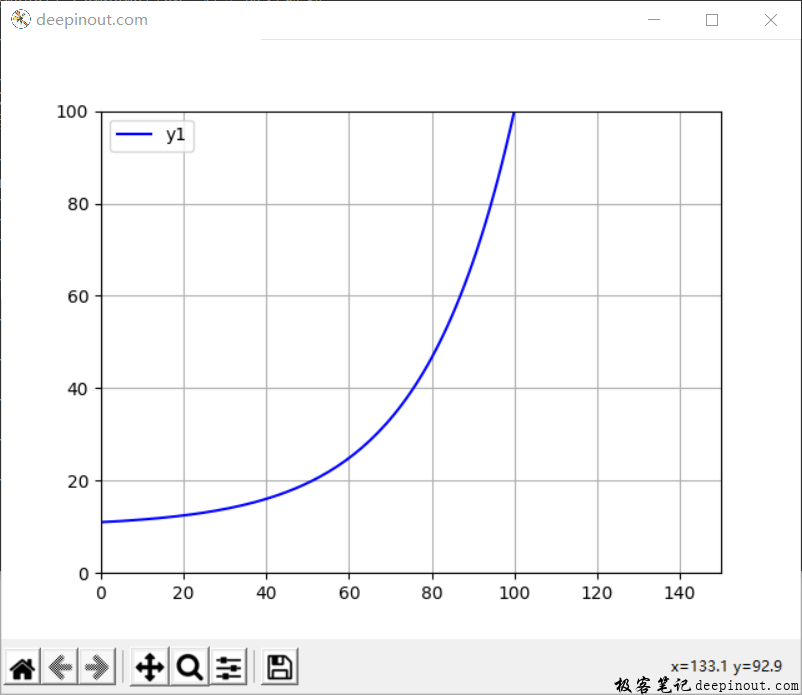# Matplotlib 显示数学曲线

plt.legend(loc = 'upper left')import numpy as np
import matplotlib.pyplot as plt

fig = plt.figure('deepinout.com')
plt.axis([0, 150, 0, 100])
plt.axis('on')
plt.grid(True)

#定义显示的数据
x = np.arange(0, 150, 1)
y1 = 10 + np.exp(0.045*x)

#显示数据
plt.plot(x, y1, 'b', label = 'y1')

#图例
plt.legend(loc = 'upper left')

plt.show()


• 回顶